Latest Banking jobs   »

# Quantitative Aptitude Quiz For RBI Grade B/ ECGC PO/ SIDBI Grade A Prelims 2022- 26th May

Directions (1-5): Study the bar chart given below and answer the following questions.
Bar chart shows the cost price of 5 different articles (A, B, C, D & E) and amount of discount allowed on these 5 articles.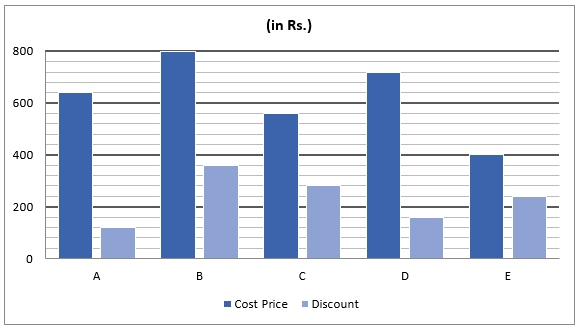Q1. Selling price of A is Rs.260 more than that of C and selling price of C is 50% more than discount allowed on C. Find marked price of A & C together is what percent of cost price of B & E together.
(a) 75%
(b) 125%
(c) 175%
(d) 100%
(e) 150%

Q2. D is marked 33⅓% above its cost price and amount of profit on B is 27.5% of marked price of B. If cost price of article – F is equal to selling price of article – B and selling price of article – F is 60% more than selling price of article – D, then find profit earned on article – F.
(a) Rs.150
(b) Rs.110
(c) Rs.70
(d) Rs.90
(e) Rs.40

Q3. Ratio of marked price of E to profit earned on E is 25 : 9 and marked price of C is equal to cost price of B. Find selling price of C & E together are how much more or less than cost price of A & B together?
(a) Rs.200
(b) Rs.120
(c) Rs.40
(d) Rs.160
(e) Rs.80

Q4. Marked price of A & B together is Rs.2000 and ratio of selling price of A to that of B is 17 : 21. Find amount of profit/loss earned by a person, if he sold 12 units of article – A and 17 units of article – B.
(a) Rs.1160
(b) Rs.1540
(c) Rs.1820
(d) Rs.820
(e) Rs.640

Q5. Profit earned on B is equal to that earned on E and selling price of B is equal to marked price of D. If selling price of D is 84% of its marked price, then find marked price of B & E together.
(a) Rs.2340
(b) Rs.2280
(c) Rs.2200
(d) Rs.2480
(e) Rs.2400

Directions (6-10): What will come in place of (?) in the following questions?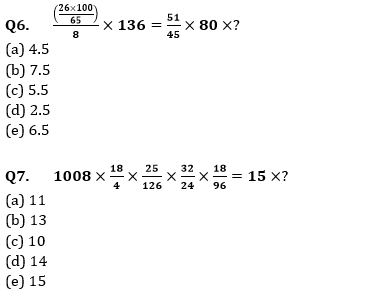Q8. (38 + 28) % of 6500 – (48 + 32) % of 4400 =?
(a) 770
(b) 730
(c) 780
(d) 810
(e) 790

Q9. (679+1589) –?% of 4000=468
(a) 55
(b) 25
(c) 45
(d) 65
(e) 15

Q10. (69×35)-(1760×150% of 380÷456)=?
(a) 185
(b) 275
(c) 245
(d) 215
(e) 305
Directions (11– 15): What value should replace question mark (?) in the following number series?

Q11. 4, 5, ?, 27, 104, 525
(a) 9
(b) 11
(c) 12
(d) 8
(e) 10

Q12. 122, 168, 226, 288, ?, 440
(a) 362
(b) 361
(c) 364
(d) 365
(e) 363

Q13. 2, ?, 30, 210, 2310, 30030
(a) 10
(b) 8
(c) 4
(d) 6
(e) 14

Q14. 45, 49, 65, 101, 165, ?
(a) 246
(b) 286
(c) 265
(d) 257
(e) 278

Q15. 33, 35, 47, ?, 133, 223
(a) 67
(b) 77
(c) 71
(d) 89
(e) 82

Solutions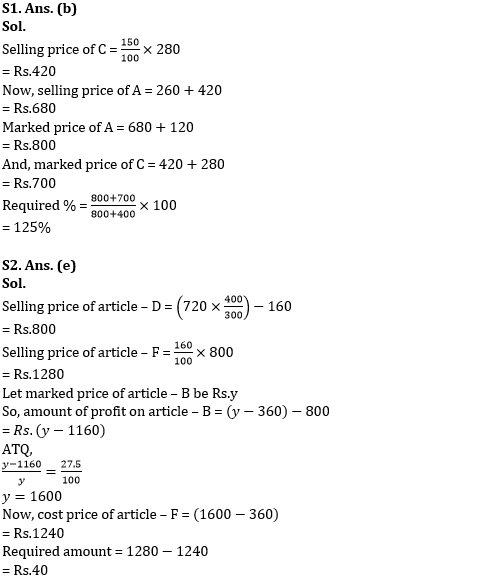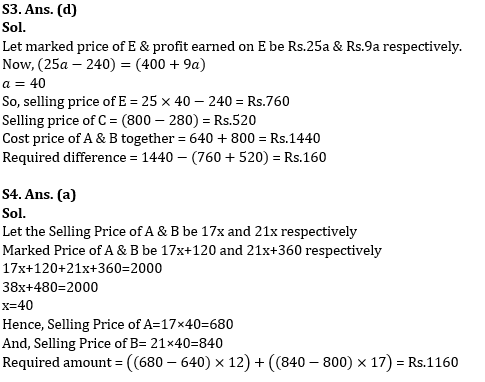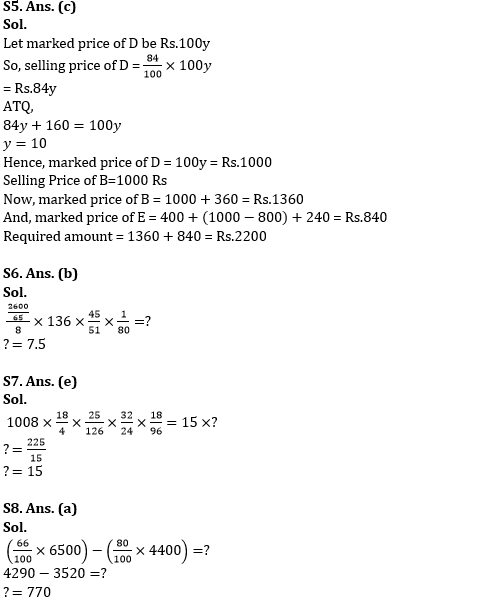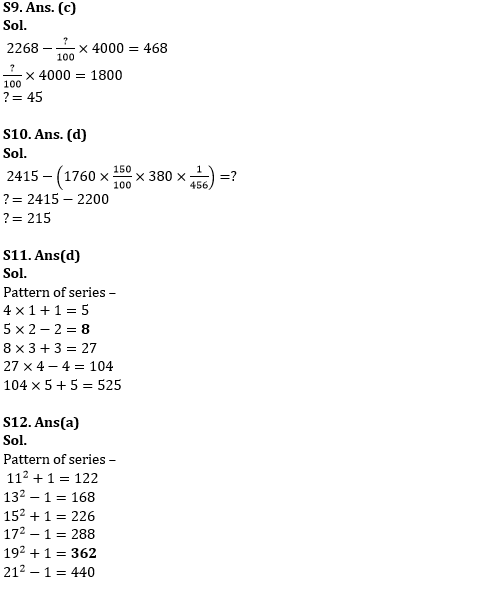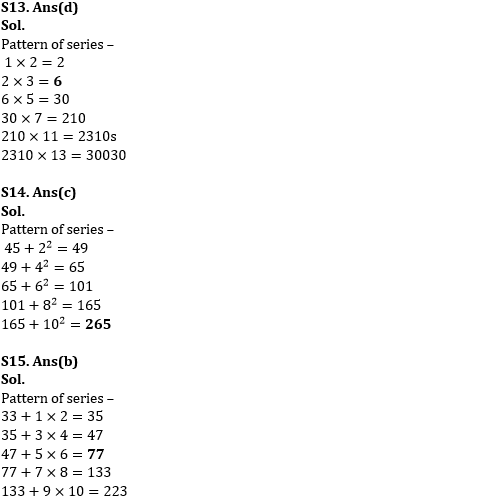#### Congratulations!